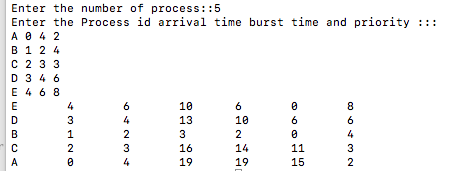# Implementation of Priority scheduling (Non Pre-emptive) algorithm using C++

In this article, we are going to learn about priority scheduling algorithm (non pre-emptive) and implementing this algorithm using C++ program.
Submitted by Aleesha Ali, on January 29, 2018

Non pre-emptive: We cannot remove a process until it completes it execution.

Scheduling criteria tells us that any algorithm is how much efficient, the main criteria of scheduling are given below:

• CPU Utilization
• Throughput
• Arrival time
• Turnaround time
• Waiting time
• Completion time
• Burst time

*Ready Queue is a queue where all the processes wait to get CPU for its execution.

CPU Utilization: The amount of time CPU is busy.

Throughput: The number of process computed per unit time.

Arrival time: The time at which the process enters into ready queue.

Turn around time: The interval between the time of submission of a process to the time of completion.

Waiting time: The total amount of the time a process spends in ready queue.

Completion time: The time at which process completes its execution.

Burst time: The time needed by CPU to completes its execution.

## Priority Scheduling Algorithm (Non Pre-emptive)

In this algorithm priority is defined by manufacture of operating system, sometimes we assume minimum number has higher priority or vice a versa.

In my algorithm I use higher number has higher priority means process having higher priority will be schedule first.

## C++ Program for Priority Algorithm (Non-preemptive)

```//Implementation of Priority(Non-Preeemptive) Using C++
#include <iostream>
#include <algorithm>
using namespace std;

typedef struct proccess
{
int at,bt,pr,ct,ta,wt;
string pro_id;

/*
artime = Arrival time,
bt = Burst time,
ct = Completion time,
ta = Turn around time,
wt = Waiting time
*/

}process;

bool compare(process a,process b)
{
return a.at<b.at;
/* This schedule will always return TRUE
if above condition comes*/

}

bool compare2(process a,process b)
{
return a.pr>b.pr;
/* This schedule will always return TRUE
if above condition comes*/

}

int main()
{
process pro;
int n,i,j;
cout<<"Enter the number of process::";
cin>>n;
cout<<"Enter the process id arrival time burst time and priority :::";

for(i=0;i<n;i++)
{
cin>>pro[i].pro_id;
cin>>pro[i].at;
cin>>pro[i].bt;
cin>>pro[i].pr;
}

sort(pro,pro+n,compare);

/*sort is a predefined funcion  defined in algorithm.h header file,
it will sort the schedulees according to their arrival time*/

pro.ct=pro.bt+pro.at;
pro.ta=pro.ct-pro.at;
pro.wt=pro.ta-pro.bt;
i=1;

while(i<n-1)
{

for(j=i;j<n;j++)
{
if(pro[j].at>pro[i-1].ct)
break;
}
sort(pro+i,pro+i+(j-i),compare2);
pro[i].ct=pro[i-1].ct+pro[i].bt;
pro[i].ta=pro[i].ct-pro[i].at;
pro[i].wt=pro[i].ta-pro[i].bt;
i++;
}
pro[i].ct=pro[i-1].ct+pro[i].bt;
pro[i].ta=pro[i].ct-pro[i].at;
pro[i].wt=pro[i].ta-pro[i].bt;

for(i=0;i<n;i++)
{
//desplaying all the values
cout<<pro[i].pro_id<<"\t"<<pro[i].at<<"\t"<<pro[i].bt<<"\t"<<pro[i].ct<<"\t"<<pro[i].ta<<"\t"<<pro[i].wt<<"\t"<<pro[i].pr;
cout<<endl;
}
return 0;
}
```

Output